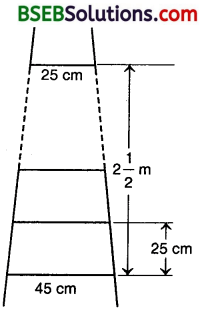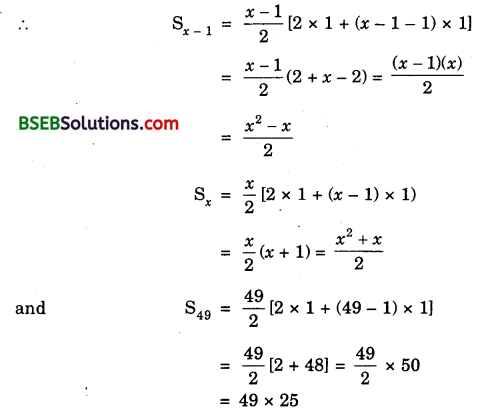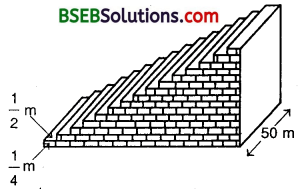# Bihar Board Class 10th Maths Solutions Chapter 5 Arithmetic Progressions Ex 5.4

Bihar Board Class 10th Maths Solutions Chapter 5 Arithmetic Progressions Ex 5.4 Textbook Questions and Answers.

## BSEB Bihar Board Class 10th Maths Solutions Chapter 5 Arithmetic Progressions Ex 5.4Question 1.
Which term of the AP : 121, 117, 113, …, is its first negative term?
Solution:
121, 117, 113, …
a = 121, d = 117 – 121 = – 4
an = a + (n – 1 )d
= 121 + in – 1) x (- 4)
= 121 – 4n + 4 = 125 – 4n
For the first negative term.
an < 0 i.e., 125 – 4n < 0
or 125 < 4n or $$\frac { 125 }{ 4 }$$ < n
or 31$$\frac { 1 }{ 4 }$$ < n n is an integer and n >31$$\frac { 1 }{ 4 }$$
∴ The first negative term is 32nd term.

Question 2.
The sum of the third and the seventh terms of an AP is 6 and their product is 8. Find the sum of first sixteen terms of the AP.
Solution:
Let the AP be a – 4d, a – 3d, a – 2d, a – d, a, a + d, a + 2d, a + 3d, …
Then, a3 = a – 2d, a7 = a – 2d
So, a3+a7 = a – 2d + a – 2d = 6
or 2a = 6 or a – 3
Also, (a – 2d)(a + 2d) = 8
So, a²-4d² = 8 or 4d² = a² – 8
or 4d² = (3)² – 8 = 9 – 8 = 1
or d² = $$\frac { 1 }{ 4 }$$ or d = ± $$\frac { 1 }{ 2 }$$
Taking d = $$\frac { 1 }{ 2 }$$Question 3.
A ladder has rungs 25 cm apart (see figure). The rungs decrease uniformly in length from 45 cm, at the bottom to 25 cm at the top. If the top and the
bottom rungs are 2$$\frac { 1 }{ 2 }$$ m apart, what is the length of the wood required for the rungs?Solution:
Number of rungs, n = $$\frac{2 \frac{1}{2} \mathrm{~m}}{25 \mathrm{~cm}}$$ = $$\frac{250 \mathrm{~cm}}{25 \mathrm{~cm}}$$ = 10
So, there are 10 rungs.
The length of the wood required for rungs
= sum of 10 rungs
= $$\frac { 10 }{ 2 }$$ [25 + 45] cm
= 5 x 70 cm
= 350 cm.

Question 4.
The houses of a row are numbered consecutively from 1 to 49. Show that there is a value of x such that the sum of the numbers of the houses preceding the * house numbered x is equal to the sum of the numbers of the houses following it. Find this value of x.
Solution:According to the question,∵ x is a counting number, so taking positive square root, x = 7 x 5 = 35.Question 5.
A small terrace at a football ground comprises 15 steps each of which is 50 m long and built of solid concrete. Each step has a rise of $$\frac { 1 }{ 4 }$$ m and a tread of $$\frac { 1 }{ 2 }$$ (see figure). Calculate the total volume of concrete required to build the terrace.Solution:
Volume of concrete required to build the first step, second step, third step, … (in m³) are
$$\frac { 1 }{ 4 }$$ x $$\frac { 1 }{ 2 }$$ x 50, ( 2 x $$\frac { 1 }{ 4 }$$ ) x $$\frac { 1 }{ 2 }$$ x 50, (3 x $$\frac { 1 }{ 4 }$$) x $$\frac { 1 }{ 2 }$$ x 50, ….
i.e., $$\frac { 50 }{ 8 }$$ x 2 x $$\frac { 50 }{ 8 }$$, 3 x $$\frac { 50 }{ 8 }$$, ….
∴ Total volume of concrete required
= [$$\frac { 50 }{ 8 }$$ + 2 x $$\frac { 50 }{ 8 }$$ + 3 x $$\frac { 50 }{ 8 }$$ + … ] m³
= $$\frac { 50 }{ 8 }$$[1 + 2 + 3 + …] m³
= $$\frac { 50 }{ 8 }$$ x $$\frac { 50 }{ 8 }$$ [2 x 1 + (15 – 1) x 1] m³ [∵ n = 15]
= $$\frac { 50 }{ 8 }$$ x $$\frac { 50 }{ 8 }$$ x 16 = 750 m³